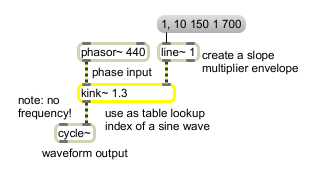# kink~

Distort a sawtooth waveform

## Description

kink~ takes phasor~ values and distorts them according to a slope factor. If the input times the slope is less than 0.5, that value is output. Otherwise, a complentary slope is used, equal to 0.5 at the same input value but equal to 1 when the input is 1. This creates a bend or "kink" in the phase waveform when the slope is not equal to 1. If you feed the output of kink~ into a cycle~, the result is that the first part (before the kink) of the wavetable is scanned much more quickly than the second part (after the kink).

## Arguments

Name Type Opt Description
slope-multiplier float opt Sets the default slope multiplier. If a signal is attached to the right inlet, this argument is ignored.

## Messages

 float slope-multiplier [float] In right inlet: Same as signal. If a signal is attached to the right inlet, float input is ignored. signal In left inlet: The input to kink~ should be a sawtooth waveform output from a phasor~ object that repeatedly goes from 0 to 1. In right inlet: The multiplier that affects the slope of the output between an output (Y) value of 0 and 0.5. After the output reaches 0.5, the waveform will increase to 1 so that the entire output moves from 0 to 1 in the same period of time as the input. A slope multiplier of 1(the default) produces no distortion Slope multipliers below 1 have a slower rise to 0.5 than the input, and slope multipliers above 1 have a faster rise to 0.5 than the input.

## Output

signal: The output of kink~ should be fed to the right inlet of cycle~ (at zero frequency) to produce a distorted sine wave (a technique known as phase distortion synthesis). As the slope multiplier in the right inlet of kink~ deviates from 1, additional harmonics are introduced into the waveform output of cycle~. If the slope multiplier is rapidly increased and then decreased using a line~, the output of cycle~ may resemble an attack portion of an instrumental sound.

## ExamplesTypical use of kink~ between phasor~ and cycle~